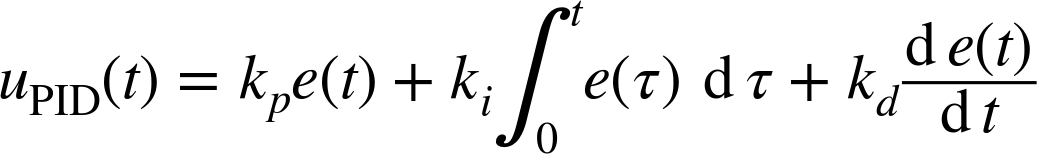## Chapter 22. PID Controllers

We encountered PID controllers already in Chapter 4. Now we take a closer look at them while using the frequency-space methods introduced in Chapter 20.

## The Transfer Function of the PID Controller

As we saw in Chapter 4, the output uPID(t) of a PID controller in terms of its input e(t) is given byNotice that uPID(t) is linear in e(t). (Both integration and differentiation are linear operations.) The linearity of the PID controller is one reason for its popularity.

We can take the Laplace transform of this expression term by term to obtain the dynamic response of a PID controller in the frequency domain. Using Table 20-1, we find without difficulty thatThe expression within brackets is the transfer function of the controller in frequency space. It has a particularly simple form—in fact, just the factors s and 1/s are often used to signify the corresponding terms (see Figure 22-1).Figure 22-1. The standard form of the three-term or PID controller.

## The Canonical Form of the PID Controller

As we have just seen, the transfer function of a PID controller isThis is the form ...

Get Feedback Control for Computer Systems now with the O’Reilly learning platform.

O’Reilly members experience live online training, plus books, videos, and digital content from nearly 200 publishers.